## Coming Soon: Maths Worksheets on Fractions, Divisibility and Multiplication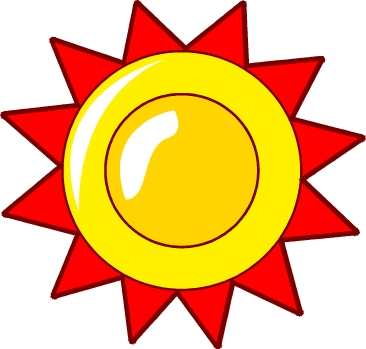Half term is coming to an end and the sun has come out at last! During the next week we will have a great range of new maths worksheets coming out to match the lovely weather. Something for all year groups here, including a measurement page for Year 1, number squares for Year 2, multiplying by 10 for year 3, and fractions for year 4. We also have a page on rules for divisibility for 9 and 10, just right for Year 5. Nothing for Year 6 this week, but they deserve a break after all those tests and are probably gearing themselves up for rounders tournaments, cricket matches, school fetes and a long break before High School.

## Maths worksheet: Long division p2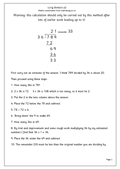The second in our series of long division pages. These are very hard for primary school children and should not be attempted until they have a good knowledge of tables, multiplying and subtraction.

Example: 789 divided by 36.

First carry out an estimate of the answer. I think 789 divided by 36 is about 20.
Then proceed using these steps:
1. How many 36s in 78?
2. 2 x 36 is 72.    3 x 36 is 108 which is too many, so it must be 2.
3. Put the 2 in the tens column above the answer.
4. Place the 72 below the 78 and subtract.
5. 78 – 72 is 6.
6. ‘Bring down’ the 9 to make 69.
7. How many 36s in 69.
8. By trial and improvement and some rough work multiplying 36 by my estimated numbers I find that 36 x 1 = 36.
9. Place the 36 under the 69 and subtract.
10. The remainder (33) must be less than the original number you are dividing by.

## Mistakes can make me feel better!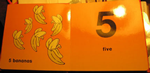With well over 500 pages and a number of games I’m sure that mathsblog has made some errors that nobody has yet spotted. If you find anything please contact me and I will do my best to correct it as soon as possible. Meanwhile, I quite enjoyed seeing this little mistake in a published book on counting – it just goes to prove that we all slip on banana skins sometimes!

Thanks to failblog for this contribution (not suitable for children).

## News: Cricket, a great game for maths!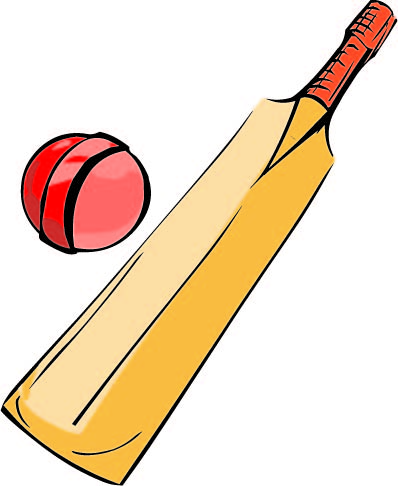Cricket – we love it!
It’s going to be one of the best summer’s ever for cricket fans. Already we are into the West Indies tour. Later we have the ICC World Twenty20 Competition, quickly followed by the latest Ashes series.
In schools, cricket is expanding again with the ‘Chance to shine’ (C2S) project, the biggest school sport-development initiative ever! And not least, cricket must be the best sports ever for maths! It abounds with maths, from adding a team’s score, working out runs per wicket, averages for batsmen and runs per over needed to win a one day game.
And don’t forget as a die has six sides it is ideal for designing simple little cricket games – just ask your year 6’s to have a go now that SAT’s are over.

## Learning tables: 6x, 7x, 8x, 9x and 10x tables pg 2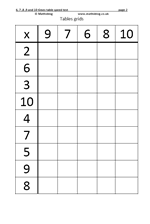This is the second page for tables 6 to 10. The idea is to fill in the grid as quickly as possible. The tens are easy, although never suggest that ‘add a naught’ will give the correct answer! The nines are interesting in that the digits have a digital root of 9 when added (1 + 8 = 9, 2 + 7 = 9 etc). The six, seven and eight times tables are probably the hardest for most children to learn.
When completing the grid various tactics can be used if the tables have not been thoroughly learned. For example, 6 x 9 is just 6 less than 6 x 10; 7 x 9 is 7 less than 7 x 10 etc.
Also if they know that 6 x 7 = 42 then use this to answer 7 x 6, which will also be 42.
However, the ultimate aim is to know tables off by heart.

6, 7, 8, 9 and 10 x tables pg 2

## Year 1 worksheets: Counting up to 20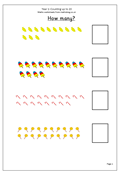Here we have a very straightforward couple of pages with up to 20 sweets to count. We work hard with children when they first start to count but then tend to neglect this area of counting on past ten. The sweets have been laid out in such a way that there are rows of ten. ‘Bright sparks’ may spot this and start at ten and just count the second row. ‘Burning hot’ sparks might do a quick subtraction with the rows that are nearly ten: it’s always interesting to watch how children set about tasks such as these.

There’s lots more for young children at urbrainy.com, which is rapidly becoming one of the best sites around for early years maths.

Count up to 20 (pg 1 and 2)

## Year 5 Maths worksheet: More equivalent fractions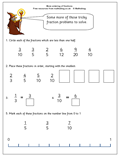Here we have some more work on understanding equivalence in fractions.

The first question looks at simple equivalence between halves, fifths, tenths etc and asks which fractions are less than a half. The quick way to work this out is to see if the top number (numerator) is less than double the bottom number (denominator) – if it is then it is less than one half.

The second question requires an understanding that a fraction such as four fifths can be changed to an equivalent fraction such as eight tenths by multiplying both the numerator and denominator by the same number (in this case 2, but it will not always be 2). All the fractions need to be changed to the same denominator which in this case would be 30.

The other questions are all aimed at improving understanding of equivalence.

More ordering fractions

## Maths worksheet: Long division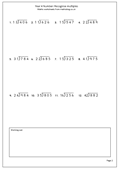Perhaps the hardest thing that children are asked to do in primary school is long division – and many children never master it, not even later in life. The main reason it is so difficult is that involves a number of stages, a good knowledge of tables and multiplication as well as subtraction. Looking at a problem such as 867 divided by 34, there are at least ten distinctive stages to go through:

34)867
First carry out an estimate of the answer. I think 867 divided by 34 is over 20 but under 30.

Then proceed using these steps:
1. How many 34s in 86?
2. 2 x 34 is 68. 3 x 34 is 102 which is too many, so it must be 2.
3. Put the 2 in the tens column above the answer.
4. Place the 68 below the 86 and subtract.
5. 86 – 68 is 18.
6. ‘Bring down’ the 7 to make 187.
7. How many 34s in 187.
8. By trial and improvement and some rough work multiplying 34 by my estimated numbers I find that 34 x 5 = 170.
9. Place the 170 under the 187 and subtract.
10. The remainder must be less than the original number you are dividing by.

## Maths worksheet: Standard subtraction of 3-digits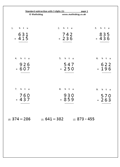Here is a basic set of subtraction questions using 3-digit numbers. They should be tackled in the standard method as described in earlier 2-digit subtraction worksheets. Notice that there are no zeros in the tens column as this can lead to particular problems and needs a set of questions all of its own.

The last three questions have been set out in a horizontal form. To answer them they should be rewritten in the standard way. Look out for mistakes where decomposition was needed but where the subtraction has been reversed eg 3 – 7 has been done as 7 – 3.

If these questions are answered correctly and in the correct method then it can be said that mastery of the subtraction method is well on its way!

Standard subtraction of 3 digits (p1)

## Coming soon: long division, equivalent fractions and tablesSome pretty meaty stuff coming during the next week or so, including the standard method of long division. Many children never master long division, with good reason, as they need to be proficient in multiplication, tables and mental subtraction to succeed. We also have some equivalent fractions for Year 5 and the standard or efficient method for subtraction for 3-digit numbers. Also coming soon is another page for practising the 6, 7, 8, 9 and 10 times tables. For younger children there are pages on Counting  up to 20.